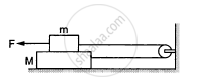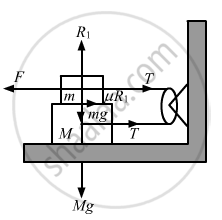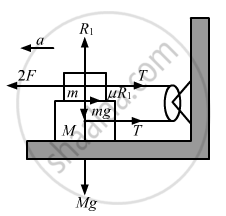Advertisement Remove all ads

# The Friction Coefficient Between the Two Blocks Shown in the Following Figure is μ but the Floor is Smooth. - Physics

Sum

The friction coefficient between the two blocks shown in the following figure is μ but the floor is smooth. (a) What maximum horizontal force F can be applied without disturbing the equilibrium of the system? (b) Suppose the horizontal force applied is double of that found in part (a). Find the accelerations of the two masses.Advertisement Remove all ads

#### SolutionFrom the free body diagrams of the two blocks, we have
R1 = mg    ...(i)
F = μR1+T    ...(ii)
T − μR1 = 0    ..(iii)
From equations (i) and (ii), we have
F − μmg = T            ...(ii)
From equations (i) and (iii), we have
T = μmg
Putting T = μmg in equation (ii), we have
F = μmg + μmg = 2μmg(b) From the free body diagram of upper block, we have
2F − T − μmg = ma       ....(i)
From the free body diagram of lower block, we have
T = Ma + μmg

Putting the value of T in (i), we get
2F − Ma − μmg − μmg = ma
Putting F = 2μmg, we get
2(2μmg) − 2μmg = a(M + m)
⇒ 4μmg − 2μmg = a(M + m)

=> a=(2mu "mg")/("M"+"m")in opposite directions.

Is there an error in this question or solution?
Advertisement Remove all ads

#### APPEARS IN

HC Verma Class 11, 12 Concepts of Physics 1
Chapter 6 Friction
Exercise | Q 24 | Page 98
Advertisement Remove all ads
Advertisement Remove all ads
Share
Notifications

View all notifications

Forgot password?